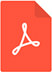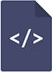# Runtime accuracy alterable approximate floating-point multipliersPDF Full Text

# Abstract

Modern systems demand high computational power within limited resources. Approximate computing is a promising approach to design arithmetic units with tight resources for error-tolerant applications such as image and signal processing and computer vision. A floating-point multiplier is one of the arithmetic units with the highest complexity in such applications. Designing a floating-point multiplier based on the approximate computing technique can reduce its complexity as well as increase performance and energy efficiency. However, an unknown error rate for upcoming input data is problematic to design appropriate approximate multipliers. The existing solution is to utilize an error estimator relying on statistical analysis. In this paper, we propose new approximate floating-point multipliers based on an accumulator and reconfigurable adders with an error estimator. Unlike previous designs, our proposed designs are able to change the levels of accuracy at runtime. Thus, we can make errors distributed more evenly. In contrast to other designs, our proposed design can maximize the performance gain since reconfigurable multipliers are able to operate two multiplications in parallel once the low accuracy mode is selected. Furthermore, we apply a simple rounding technique to approximate floating-point multipliers for additional improvement. Our simulation results reveal that our new method can reduce area by 70.98% when error tolerance margin of our target application is 5%, and when its error tolerance margin is 3%, our rounding enhanced simple adders-based approximate multiplier can save area by 65.9%, and our reconfigurable adder-based approximate multiplier with rounding can save the average delay and energy by 54.95% and 46.67% respectively compared to an exact multiplier.

# Keywords

approximate computing, multiplier, error tolerance, estimator, reconfigurable, floating-point